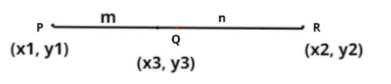QUESTION

# If points P (-7, 1), Q (x, 4) and R (3, 4) are collinear and PQ=QR. Find the values of x and y.

Hint – In this question use the concept that the ratio in which point Q divides the line segment formed by P and R will be in the ratio 1: 1 as the points are collinear.Let P = (x1, y1) = (-7, 1)
Q = (x3, y3) = (x, 4)
R = (x2, y2) = (3, y)
Now it is given that these three points are collinear and PQ = QR therefore point Q divide the line PR in the ratio (1 : 1) so by section formula coordinates of Q are
$\Rightarrow {x_3} = \dfrac{{m{x_2} + n{x_1}}}{{m + n}},{y_3} = \dfrac{{m{y_2} + n{y_1}}}{{m + n}}$
Here m = 1 and n = 1.
So substitute all the values in the above equation we have,
$\Rightarrow x = \dfrac{{3 + \left( { - 7} \right)}}{{1 + 1}},4 = \dfrac{{y + 1}}{{1 + 1}}$
So on simplifying we have,
$\Rightarrow x = \dfrac{{ - 4}}{2},8 = y + 1$
$\Rightarrow x = - 2,y = \left( {8 - 1} \right) = 7$
Therefore x = -2 and y = 7.
So this is the required answer.

Note – Points are said to be collinear if and only if they belong to the same line. It is advised to remember the section formula and another method to solve this problem would have been the concept that if points are collinear then slopes of two pairs of points onto that line should be equal.